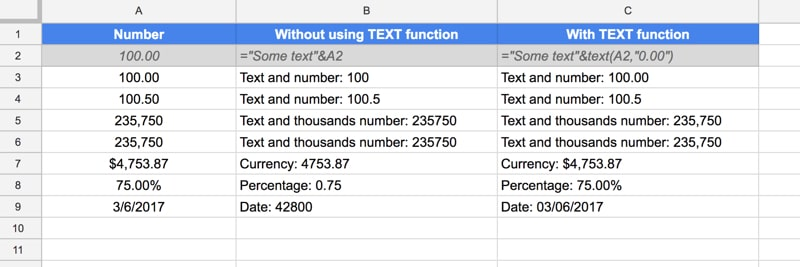# How To Combine Text And Numbers In Google Sheets

This tutorial will show you how to combine text and numbers in Google Sheets, and keep the the correct number formatting in place.

Combining text and numbers is common in spreadsheets, for example when you want to add some context:

e.g. `Total: \$5,250`
e.g. `Discount: 35.5%`

However, if you try to combine text with numbers (or currencies/percentages/dates) directly in a cell in Google Sheets, you’ll notice the numbers loses any formatting, which will make the number harder to read or even less meaningful:

e.g. `Total: 5250`
e.g. `Discount: 0.355`

You can use the TEXT function to fix this, and apply formatting to numbers that are displayed with text in a cell.

## Combine Text And Numbers In Google Sheets

To combine text, in a cell or denoted by quotes `"Text"`, and numbers, use the TEXT function, as shown in these examples.

For these examples, I’m assuming I have numbers, currency numbers, percentages or dates in column A:### What’s the formula?

With numbers in column A, then you can combine text and numbers with:

`="Text and number: "&text(A1,"0.00")`
`="Text and number: "&text(A2,"#.##")`

What’s the difference?

The 0 forces your cell to display zeros if a number has fewer digits than the format specifies, whereas the # does not force the display of zeros.

So `TEXT(19.9,"000.00")` displays `019.90`, whereas `TEXT(19.9,"###.##")` displays `19.9`.

For a deeper look at number formatting in Google Sheets, read my Google Sheets custom number format tutorial.

Thousands separator

If your number is in the thousands, and you want to show the thousand separator comma, simply add that into the format section of the text formula. It works the same for the “0” or “#” notation:

`="Text and thousands number: "&text(A3,"0,000")`
`="Text and thousands number: "&text(A3,"#,###")`

Currency

Combining text and currency is easy enough, now you know how to format numbers. Simply add the currency symbol to the front of the number and it will show in your text:

`="Currency: "&text(A4,"\$0,000.00")`

Percentages

Combining text and percentages:

`="Percentage: "&text(A5,"0.00%")`

Dates

Combining text and dates:

`="Date: "&text(A6,"mm/dd/yyyy")`

## One thought on “How To Combine Text And Numbers In Google Sheets”

1.Gaurang says:

Hello

What if A has any value text or number and I want to add comment “finish” on B